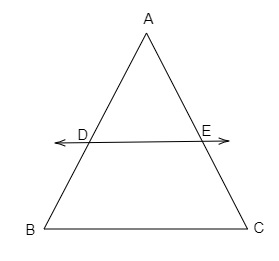# If $D$ and $E$ are points on sides $AB$ and $AC$ respectively of a $\triangle ABC$ such that $DE \parallel BC$ and $BD = CE$. Prove that $\triangle ABC$ is isosceles.

Given:

$D$ and $E$ are points on sides $AB$ and $AC$ respectively of a $\triangle ABC$. $DE \parallel BC$ and $BD = CE$.

To do:

We have to prove that $\triangle ABC$ is isosceles.

Solution:From the figure,

$DE \parallel BC$

By basic proportionality theorem,

$\frac{AD}{DB}=\frac{AE}{EC}$

$\Rightarrow \frac{AD}{DB}=\frac{AE}{DB}$   (Since $BD=CE$)

$\Rightarrow AD=AE$

Adding $DB$ on both sides, we get,

$\Rightarrow AD+DB=AE+DB$

$\Rightarrow AD+DB=AE+EC$   (Since $BD=CE$)

$\Rightarrow AB=AC$

$\Rightarrow \triangle ABC$ is isosceles.

Hence proved.